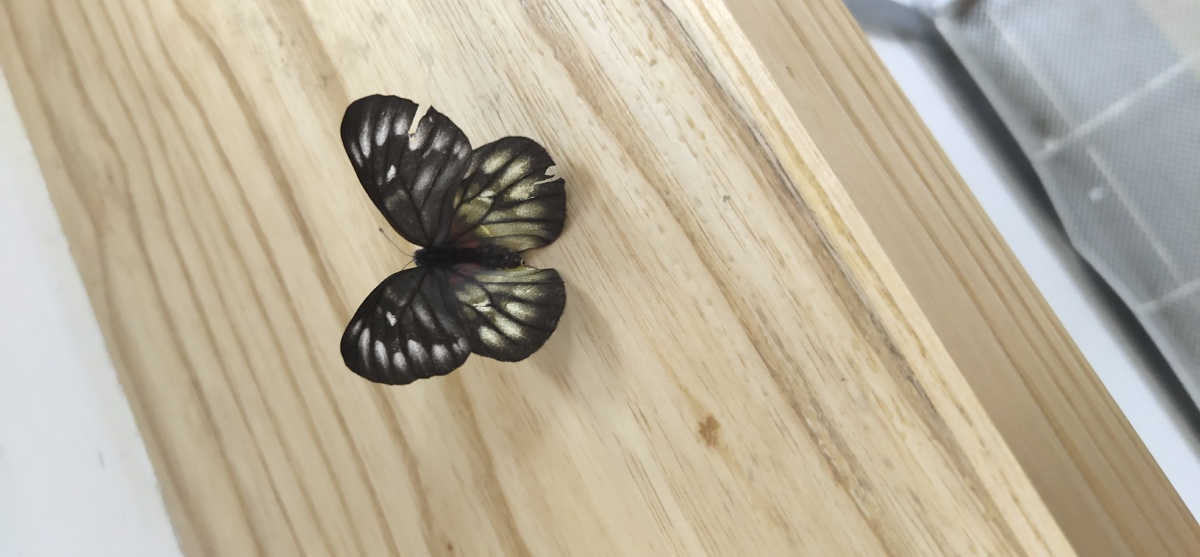# 非空有限集合子集和真子集的个数规律

、{a}、{b}、{c}、{a,b}、{a,c}，{b,c}、{a，b,c}=2

1、子集个数：：∅

、{a}、{b}、{c}、{d}、{a,b}、{a,c}，{a,d}、{b,c}、{b,d}、{c,d}、{a,b,c}、{a,b,d}、{a,c,d}{b,c,d}、{a,b,c,d}=2

1、子集个数：：∅

、{a}、{b}、{c}、{d}、{d}、{a,b}、{a,c}，{a,d}、{a,e}、{b,c}、{b,d}、{b,e}、{c,d}、{c,e}，……，{a,b,c,d,e}=2

1、n个元素的集合的子集个数是：